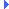FD.io VPP  v20.05-21-gb1500e9ff Vector Packet Processing
bitops.h File ReferenceInclude dependency graph for bitops.h:This graph shows which files directly or indirectly include this file:

Go to the source code of this file.

## Data Structures

struct  compress_main_t

## Functions

static uword count_set_bits (uword x)

static void compress_init (compress_main_t *cm, uword mask)

static uword compress_bits (compress_main_t *cm, uword x)

static uword rotate_left (uword x, uword i)

static uword rotate_right (uword x, uword i)

static uword next_with_same_number_of_set_bits (uword x)

## ◆ foreach_set_bit

 #define foreach_set_bit ( var, mask, body )
Value:
do { \
uword _foreach_set_bit_f_##var; \
while (_foreach_set_bit_m_##var != 0) \
{ \
_foreach_set_bit_f_##var = first_set (_foreach_set_bit_m_##var); \
_foreach_set_bit_m_##var ^= _foreach_set_bit_f_##var; \
(var) = min_log2 (_foreach_set_bit_f_##var); \
do { body; } while (0); \
} \
} while (0)
static uword min_log2(uword x)
Definition: clib.h:159
static uword first_set(uword x)
Definition: clib.h:268

Definition at line 166 of file bitops.h.

## ◆ compress_bits()

 static uword compress_bits ( compress_main_t * cm, uword x )
inlinestatic

Definition at line 118 of file bitops.h.

## ◆ compress_init()

 static void compress_init ( compress_main_t * cm, uword mask )
inlinestatic

Definition at line 91 of file bitops.h.

## ◆ count_set_bits()

 static uword count_set_bits ( uword x )
inlinestatic

Definition at line 45 of file bitops.h.Here is the caller graph for this function:

## ◆ next_with_same_number_of_set_bits()

 static uword next_with_same_number_of_set_bits ( uword x )
inlinestatic

Definition at line 156 of file bitops.h.Here is the call graph for this function:

## ◆ rotate_left()

 static uword rotate_left ( uword x, uword i )
inlinestatic

Definition at line 142 of file bitops.h.Here is the caller graph for this function:

## ◆ rotate_right()

 static uword rotate_right ( uword x, uword i )
inlinestatic

Definition at line 148 of file bitops.h.